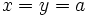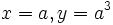# Alternative implies powers up to the fifth are well-defined

This article gives the statement and possibly, proof, of an implication relation between two magma properties. That is, it states that every magma satisfying the first magma property (i.e., alternative magma) must also satisfy the second magma property (i.e., magma in which powers up to the fifth are well-defined)
View all magma property implications | View all magma property non-implications
Get more facts about alternative magma|Get more facts about magma in which powers up to the fifth are well-defined

## Statement

Suppose$(S,*)$ is an alternative magma, i.e., it satisfies the following two identities:$x * (x * y) = (x * x) * y \ \forall \ x,y \in S$

and$x * (y * y) = (x * y) * y \ \forall \ x,y \in S$

These are respectively termed the left-alternative law and right-alternative law.

Then cubes, fourth powers, and fifth powers are well-defined in$S$. In other words, if$x^2$ denotes$x*x$, we have the following for all$a \in S$:

1. Cubes are well-defined:$a^2 * a = a * a^2$, and this is denoted as$a^3$.
2. Fourth powers are well-defined:$a^3 * a = a^2 * a^2 = a * a^3$, and this is denoted as$a^4$.
3. Fifth powers are well-defined:$a^4 * a = a^3 * a^2 = a^2 * a^3 = a * a^4$ and this is denoted as$a^5$.

## Proof

As above, we assume$(S,*)$ is an alternative magma and$a$ is an arbitrary element of$S$.

### Cubes are well-defined

To prove:$a * a^2 = a^2 * a$.

Proof: This follows from either of the alternative laws, setting$x = y = a$.

### Fourth powers are well-definedWe note that even after removing the edges labeled "Flex", the graph is still connected. This shows that fourth powers are well-defined

To prove:$a^3 * a = a^2 * a^2 = a * a^3$.

Proof: We first show that$a^3 * a = a^2 * a^2$. Consider:$\! a^3 * a = (a^2 * a) * a$

By the right-alternative law, setting$x = a^2, y = a$, we obtain that:$\! a^3 * a = a^2 * (a * a) = a^2 * a^2$

We now show that$a^2 * a^2 = a * a^3$. We have:$\! a^2 * a^2 = (a * a) * a^2$

By the left-alternative law, setting$x = a, y = a^2$, we obtain:$\! a^2 * a^2 = a * (a * a^2) = a * a^3$.

Thus, we have shown that all three expressions are equal.

### Fifth powers are well-definedWe note that even after removing the edges labeled "Flex", the graph is still connected. This proves that fifth powers are well-defined.

To prove:$a^4 * a = a^3 * a^2 = a^2 * a^3 = a * a^4$.

Proof:

We first show that$a^4 * a = a^3 * a^2$. Note that:$\! a^4 * a = (a^3 * a) * a = a^3 * (a * a) = a^3 * a^2$

where, in an intermediate step, we used the right-alternative law with$x = a^3, y = a$.

We next show that$a^3 * a^2 = a * a^4$. Note that:$\! a^3 * a^2 = (a * a^2) * a^2 = a * (a^2 * a^2) = a * a^4$

where, in an intermediate step, we used the right-alternative law with$x = a, y = a^2$.

We finally show that$a * a^4 = a^2 * a^3$. Note that:$\! a * a^4 = a * (a * a^3) = (a * a) * a^3 = a^2 * a^3$

where, in an intermediate step, we used the left-alternative law with$x = a, y = a^3$.

This completes the proof.